## Wednesday, August 8, 2007

### Irodov Problem 1.70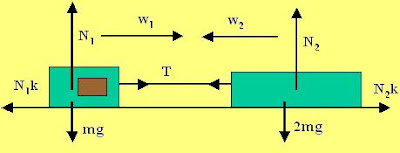Let tension in the string joining the two bodies be T.

Forces on the bar:
First let us consider the forces acting on the bar. Along the vertical direction there are two forces, the normal reaction from the plane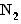, and the force of gravity 2mg. There is no component of acceleration for the bar along this direction. Thus, we can write,Now, let us consider the forces in the vertical direction. There are two forces acting on the bar in the horizontal direction, i) the tension in the string T and ii) the force of frictionopposing the motion of the bar. The acceleration of the block is. Hence we have,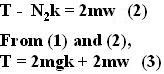Forces on the motor block:
In the vertical direction there are two forces acting on the motor block, i) the normal reaction from the planeand ii) the force of gravity mg. There is no acceleration of the motor block along the vertical direction and hence we have,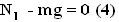In the horizontal direction, there are again two forces acting on the block, i) the tension in the string T and ii) force of frictionthat opposes the motion of the block . Let the acceleration of the motor block be. Then we have,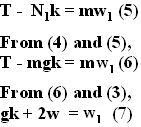The net relative acceleration (rate of approach) between the motor block and the bar is the sum of their individual accelerations with respect to the plane given by,Let the time taken for the two bodies to collide be t, then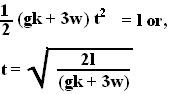#### 1 comment:Anonymous said...

thanks man i was not taking the net acceleration into account